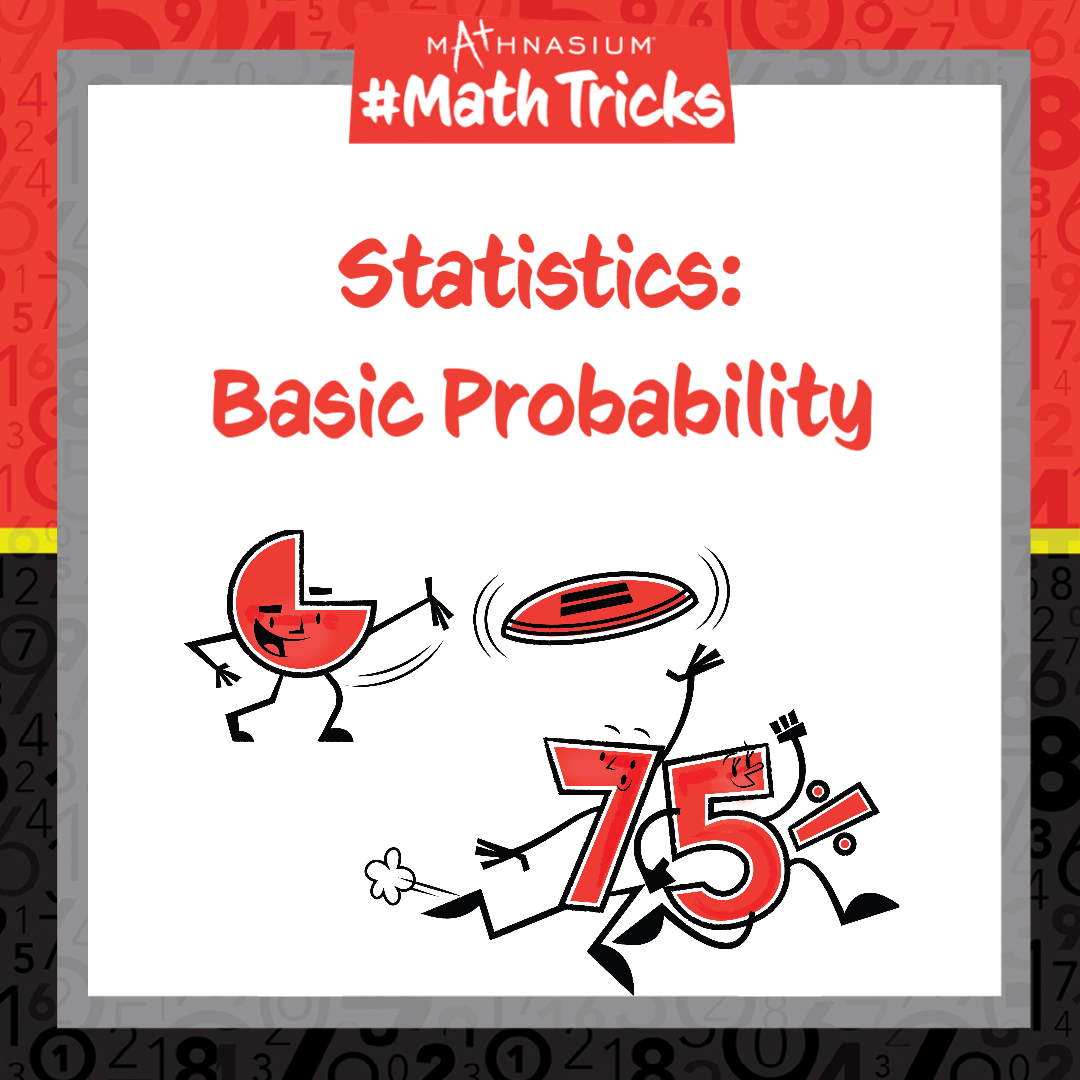877-601-6284
Get Started by Finding a Local Center

# Mathnasium #MathTricks: Probability (Part 1)

Sep 28, 2022Welcome to Mathnasium’s Math Tricks series. Today we are calculating basic probability.

Probability is the chance or likelihood that something will happen. To calculate the basic probability of something happening, we make a fraction of the number of ways an event can happen (the “favorable” outcomes) out of the total number of “possible” outcomes. Probability can also be represented as a decimal or percent.

The likelihood that something will happen falls between 0 or 0%, (impossible events), and 1 or 100%, (certain events). All other probabilities fall in between 0 and 1.Follow the examples below to find the basic probabilities of the events.

##### Example 1: A bag contains 3 red, 4 white, and 6 blue marbles. If 1 marble is pulled from the bag at random, what is the probability that the marble is red OR white?

Step 1: Find the total number of possible outcomes.
There is a total of: 3 red marbles + 4 white marbles + 6 blue marbles = 13 marbles.

Step 2: Find the total number of favorable outcomes.
The favorable outcome is pulling a red OR white marble from the bag at random.
The total number of favorable outcomes is: 3 red + 4 white marbles = 7 marbles.

##### Example 2: If a blue marble was pulled from the same bag and not replaced, what is the probability of drawing another blue marble?

Step 1: Find the total number of possible outcomes.
Since a blue marble was pulled from the same bag, there are now: 13 - 1 = 12 marbles.

Step 2: Find the total number of favorable outcomes.
The favorable outcome is drawing another blue marble.
The total number of favorable outcomes is: 6 - 1 = 5 blue marbles.

If you missed this, or any of our other Math Tricks videos, check them out on our YouTube channel!

## SEE HOW MATHNASIUM WORKS FOR YOUR SITUATION

### My Child is:## OUR METHOD WORKS

Mathnasium meets your child where they are and helps them with the customized program they need, for any level of mathematics.Early LearnersElementary SchoolMiddle SchoolHigh School